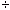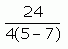SEARCH HOMEMath Central Quandaries & QueriesSubject: bedmas with integers Name: joanne Who are you: Teacher getting 2 different answers for 24divided by 4(5-7) i can't find the divide sign on this keyboard getting -12 or -3 can you please explain the thinking behind each?We have three replies for you.

Hi Joanne,

You have

244(5-7).

First you should perform the operation inside that parentheses to get

244(-2).

In bedmas division comes before multiplication and 244 = 6 so your expression becomes

6(-2) which is -12.

Penny

Hi Joanne.

We usually use the forward slash to represent division on the keyboard: /

24/4(5-7)

There is an implied multiplication between the 3 and the left
parenthesis, so using bedmas:

24/4(5-7)
= 24/4x(-2)
= 6x(-2)
= -12

However, if you meant that you have a fraction of the formthen that is in fact written as 24/(4(5-7)), so bedmas tells us:

24/(4x(5-7))
= 24/(4x(-2))
= 24/(-8)
= -3

Hope this explanation helps,
Stephen La Rocque.

Hi Joanne,

You're starting with:
24 / 4 * (5-7)
(I put the multiplication symbol in for clarity, though you don't need to write it if you don't want to)

The order of operations say you take care of things inside the parentheses first, so next you have:
= 24 / 4 (-2)

Now the only operations left are division and multiplication. These should be done in the order that they appear, from left to right. The first operation we encounter in the expression is 24/4, so do that one first:
=6 * (-2)
= -12

One way that you may have gotten -3 as the answer is if you looked at the 24 as being divided by both the 4 and the (5-7) in the original expression. This is would be an incorrect interpretation of the original expression. On the other hand, if you started with the following expression, you would get -3, because the extra parentheses tell you 24 will be divided by 4*(5-7):

24 / [ 4 * (5-7) ]
= 24 / [4 * (-2)]
= 24 / -8
= -3

Hope this helps,
HaleyMath Central is supported by the University of Regina and The Pacific Institute for the Mathematical Sciences.